# Problem-1 (Cost structure, target profit analysis, CM ratio, break-even analysis)

Zoltrixound company manufactures high quality speakers for desktop and laptop computers. Last month Zoltrixound suffered a loss of \$18,000. The income statement for the last month is as follows: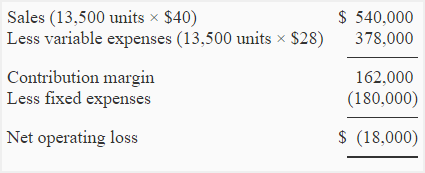Required:

1. Compute the break-even point and contribution margin ratio (CM ratio) of Zoltrixound company?
2. Sales department feels that if monthly advertising budget is increased by \$16,000, the sales will be increased by 3,500 units. Show the effect of these changes assuming the selling price remains unchanged.
3. If sales price is reduced by 20% and monthly advertising expenses are increased by \$70,000, the unit sales are expected to increase by 100%. Show the effect of this change by preparing a new income statement of Zoltrixound company.
4. The Zoltrixound wants to make the packing of  its product more attractive. The new packing would increase cost by \$1.20 per unit. Assuming no other changes, compute the number of units to be sold to earn a net operating income of \$9,000.
5. The company is planning to purchase a new machine. The installation of  new machine will increase fixed cost by \$236,000 and decrease unit variable expenses by 50%.
(a). Compute the CM ratio and break-even point if the new machine is installed.
(b). Company expects a sale of 20,000 units for the next month. Prepare two income statement, one assuming that the machine is not installed and one assuming that it is installed.
(c) Should the company install new machine. Give your recommendations.

## Solution:

### (1) Computation of CM ratio and break-even point:

a. Contribution margin ratio = Contribution margin/Net sales

= \$162,000/\$540,000

= 0.30 or 30%

b. Break-even point in units = Fixed expenses/Contribution margin per unit

= \$180,000/\$12*

= 15,000 units

*\$162,000/13,500 units

Break-even point in dollars = Break-even point in units × Sale price

=  15,000 units × \$40

= \$600,000

### (2) Effect of increase in advertising budget by \$16,000 and sales by \$140,000: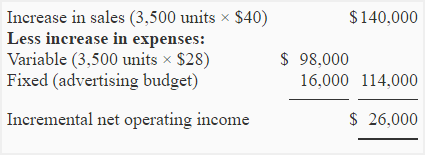If monthly advertising budget is increased by \$16,000 and sales revenues is increased by \$140,000, the company will earn a profit of \$8,000 (\$26,000 – \$18,000) rather than suffering a loss.

### (3) Effect of reduction in sales price by 20%, increase in monthly advertising budget by \$70,000 and increase in unit sales by 100%: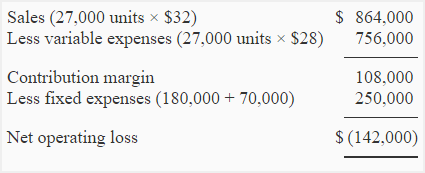### (4) Computation of the number of units to be sold to earn \$9,000:

Unit sale for target profit = (Fixed expenses + Target profit)/Contribution margin per unit

= (\$180,000 + \$9,000) / \$10.80*

= 17,500 Units

*The new packing will increase variable expenses from \$28 per unit to \$29.20 per unit and reduce the contribution margin from \$12 per unit to \$10.80 per unit.

[\$40 – (\$28 + \$1.20)] = \$10.80

### (5). Installation of new machine:

a. Contribution margin ratio and break-even point if new machine is installed:

i. Contribution margin ratio = Contribution margin/Net sales

= \$351,000/\$540,000

= 0.65 or 65%

ii. Break-even point in units = Fixed expenses/Contribution margin per unit

= \$416,000/\$26*

= 16,000 units

*Variable expenses have decreased from \$28 to \$14 (50% reduction) therefore the contribution margin would increase from \$12 per unit to \$26 per unit (\$40 – \$14).

Break-even point in dollars = Break-even point in units × Sale price

= 16,000 units × \$40

= \$640,000

(b). Income statement with and without installing new machine:

If new machine is not installed: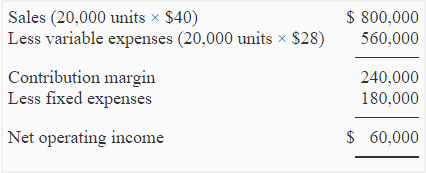If new machine is installed: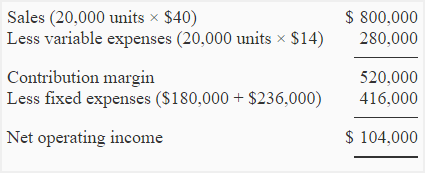c. Decision about installing new machine:

If only the monthly net operating income is considered, Zoltrixound should purchase and install the new machine because it will generate more net operating income. At 20,000 units per month, variable expenses will decrease by \$280,000 (20,000 units × \$14) and monthly fixed expenses will increase by only \$236,000. The saving in variable expenses is therefore greater than the increase in fixed expenses.

A D V E R T I S E M E N T
3 Comments on Problem-1 (Cost structure, target profit analysis, CM ratio, break-even analysis)
1.Edyta

Sales (27,000 units × \$32 ) is not \$972,000, it is 864,000.

2.Accounting for Management

Corrected.

3.TENY

any one can help me cuz i dnt understand break even